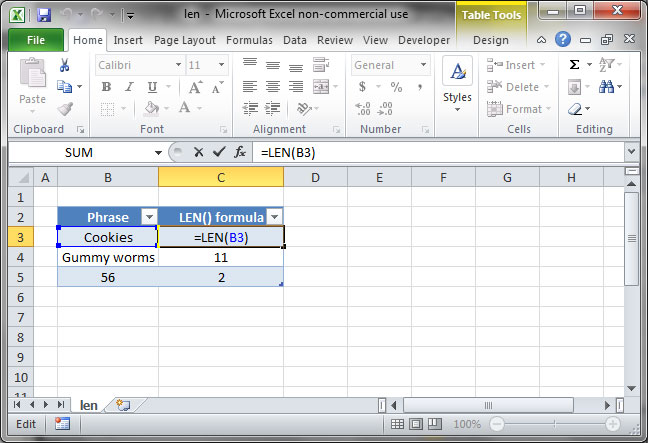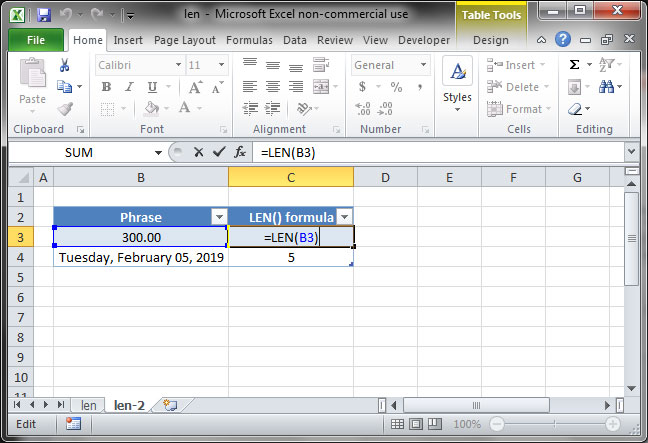# Using the LEN function

The LEN function is one of Excel's most useful string-manipulation tools. This simple formula outputs the length of any given input. Use it like this:

`=LEN(text)`

LEN also works when you call it on individual cells. Take a look at the following spreadsheet, in which we have called the LEN function on three separate cells:`=LEN(B3)Output: 7`
`=LEN(B4)Output: 11`
`=LEN(B5)Output: 2`

## Cell formatting complications

It’s important to note that the LEN function calculates the length of inputs in their raw form; formatting is ignored.

In the following example, the function returns a value of 3 when pointed to cell B3, despite the fact that the text in cell B3 is 300.00. This is because the actual value in the cell is 300; the decimal point and extra zeroes are displayed due to number formatting, but are ignored by the LEN function.`=LEN(B3)Output: 3`

## Save an hour of work a day with these 5 advanced Excel tricks

Work smarter, not harder. Sign up for our 5-day mini-course to receive must-learn lessons on getting Excel to do your work for you.

• How to create beautiful table formatting instantly...
• Why to rethink the way you do VLOOKUPs...
• Plus, we'll reveal why you shouldn't use PivotTables and what to use instead...